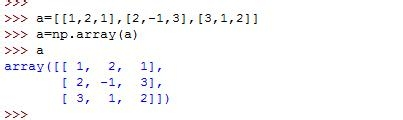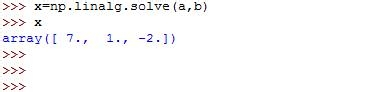• 首先看一下我们要解的方程，将这个方程格式调整好，按照x-y-z-常数项的顺序排列• 将未知数的系数写下来，排列成一个矩阵a，如下• 常数项构成一个一维数组（向量）• 使用linalg.solve方法解方程，参数a指的是系数矩阵，参数b指的是常数项矩阵• 我们得到的解对不对呢？使用点乘的方法可以验证一下，系数乘以未知数可以得到常数项• 今天用到的所有代码如下

• a=[[1,2,1],[2,-1,3],[3,1,2]]

• a=np.array(a)

• a

• array([[ 1, 2, 1],

• [ 2, -1, 3],

• [ 3, 1, 2]])

• b=[7,7,18]

• b=np.array(b)

• b

• array([ 7, 7, 18])

• x=np.solve(a,b)

• Traceback (most recent call last):

• File “<pyshell#31>”, line 1, in

• x=np.solve(a,b)

• AttributeError: ‘module’ object has no attribute ‘solve’

• x=np.linalg.solve(a,b)

• x

• array([ 7., 1., -2.])

• np.dot(a,x)

• array([ 7., 7., 18.])